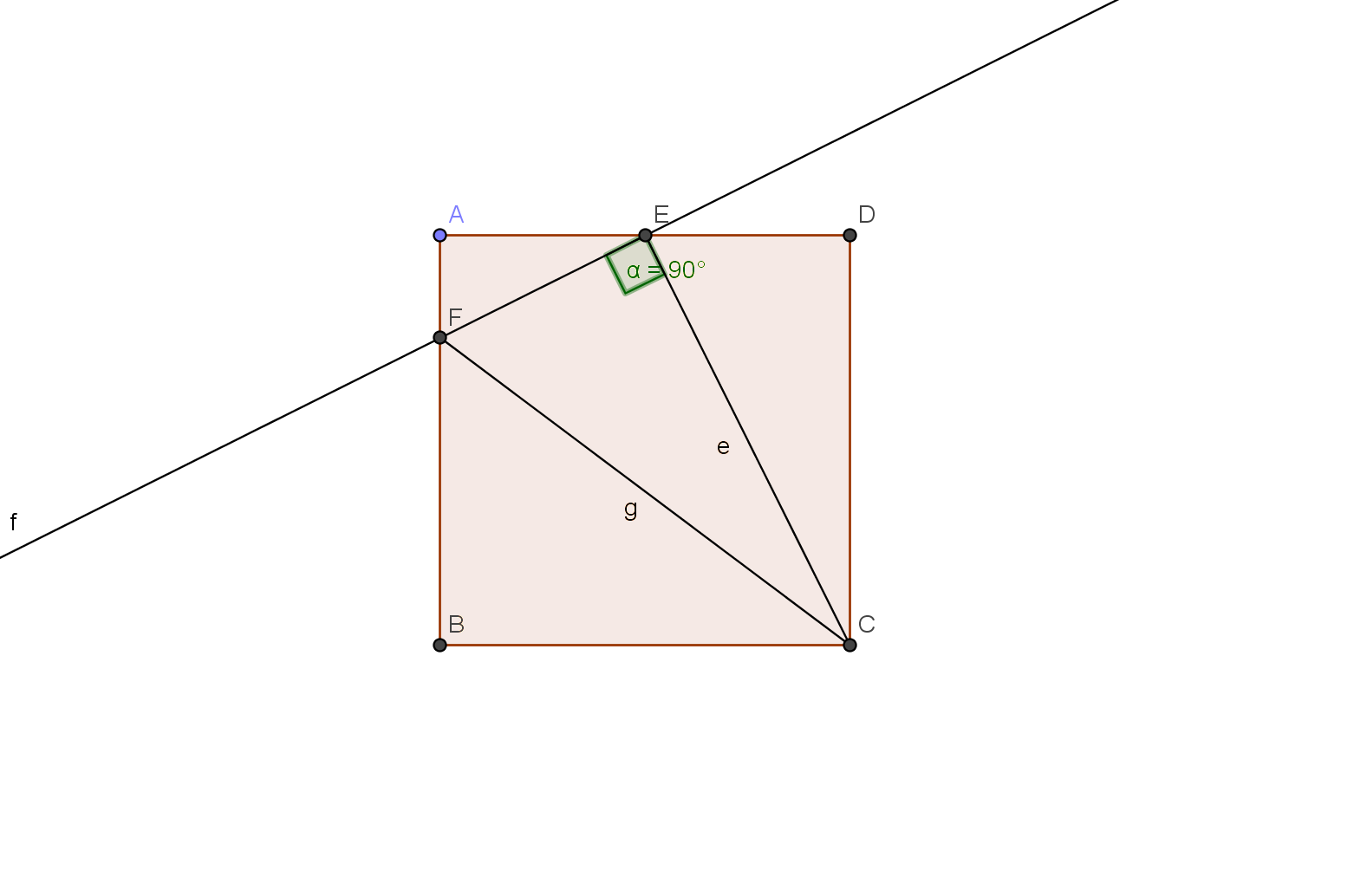# Awesome geometry - 9

Geometry Level 3In square ABCD ,

Point E is the mid point of AD.

Join CE.

Let Point F be on AB such that angle CEF measures 90 .

If measure of angle CED is 63.43 ,

Find the measure of angle ECF. Give your answer to correct 2 decimals.

×